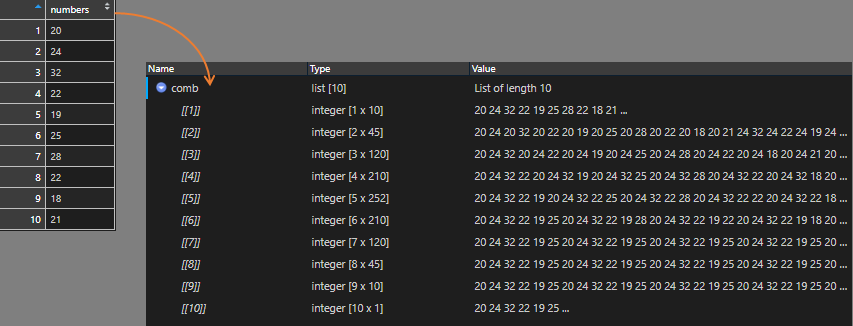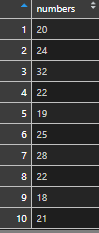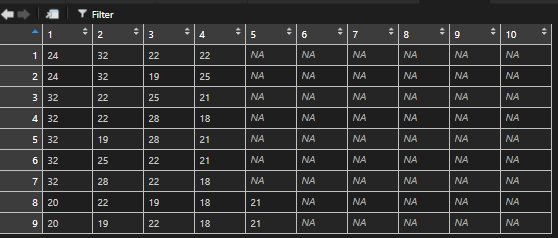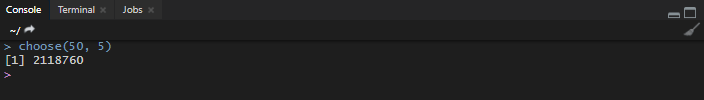# How to combine numbers and find sums with RIn this post, I will explain the approach on how to combine numbers with R to find out which of them makes a certain sum. First of all, we will generate all possible number combinations from the vector or data frame column. That will give us multiple matrices. Then we will combine them in a single data frame and select rows that contain the sum that we need.

The full code is at the end of this post.

Here is a data frame with one column containing ten numbers.
The question is – which of those numbers gives a sum of 100 in any possible combination.

```# sample data frame
df <- structure(list(numbers = c(
20L, 24L, 32L, 22L, 19L, 25L, 28L,
22L, 18L, 21L
)),
class = "data.frame",
row.names = c(NA, -10L))```By using lapply, it is possible to generate multiple matrices for each of the combinations that are made with function combn. The first matrix is with the 1 number combinations, second with 2 numbers combinations, third with 3 number combinations, etc.

```require(plyr)
require(dplyr)

# count of numbers
n <- nrow(df)

# create all possible combinations to get sum
comb <- lapply(1:n, function(x) combn(df\$numbers, x))```To combine multiple matrices, I used function rbind.fill.matrix and, as you see, there is a function t to transpose every matrix before binding together. After combining all, it is easier to find and filter certain combinations.

```# transpose each matrix and append them together
all_comb <- rbind.fill.matrix(sapply(comb, function(x) t(x)))```When matrices are combined, it is possible to subset number combinations that are equal to 100.

```# subset all rows from matrix that contains desired sum
result <- subset(all_comb, rowSums(all_comb, na.rm = T) == 100)```There might be a situation when some of the columns contain only NA values. That is normal because, after combining multiple matrices with different sizes, the number of columns is defined by the largest matrix.

#### Here is how to remove columns that contain only NA.

```# get rid of columns that contains only NA
result <- as.data.frame(result)
result <- result[colSums(!is.na(result)) > 0]```You can easily copy data from Excel to R and results back to Excel. Take a look at the post on how to do that in RStudio.

## Dealing with a large number of combinations

If you are looking for a certain sum, the first thing to consider – do you have a clue what might be the maximum of number combinations? For example, if you have 50 numbers, then you can calculate how many there are unique combinations that contain 5 of them.With the larger amount of numbers, you can run out of memory. If you have no idea if you need to combine everything, try to start with the small amount and try to increment that.
For example, 3 number combinations like this.

```comb <- combn(df\$numbers, 3)
View(comb)```

#### Is there room for speed improvement?

Most likely – yes. Matrix object for me worked faster then data.table. You can adjust this script and run it from the Windows command line like this.

You may also try expand.grid to combine numbers with R. For example, 3 number combinations.

```# another way to make combinations
# in this example - 3 number combinations
comb2 <- expand.grid(df\$numbers,df\$numbers,df\$numbers)```

#### Here is all the code.

```# sample data frame
df <- structure(list(numbers = c(
20L, 24L, 32L, 22L, 19L, 25L, 28L,
22L, 18L, 21L
)),
class = "data.frame",
row.names = c(NA, -10L))

require(plyr)
require(dplyr)

# count of numbers
n <- nrow(df)

# create all possible combinations to get sum
comb <- lapply(1:n, function(x) combn(df\$numbers, x))

# transpose each matrix and append them together
all_comb <- rbind.fill.matrix(sapply(comb, function(x) t(x)))

# subset all rows from matrix that contains desired sum
result <- subset(all_comb, rowSums(all_comb, na.rm = T) == 100)

# get rid of columns that contains only NA
result <- as.data.frame(result)
result <- result[colSums(!is.na(result)) > 0]

# another way to make combinations
# in this example - 3 number combinations
comb2 <- expand.grid(df\$numbers,df\$numbers,df\$numbers)```